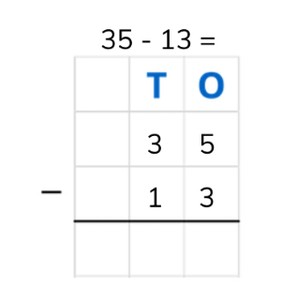Subtraction to 1,000 using standard algorithm

# Subtraction to 1,000 using standard algorithm

Students learn to subtract numbers to 1,000 using the standard algorithm.

No account needed.8,000 schools use Gynzy92,000 teachers use Gynzy1,600,000 students use Gynzy

## General

Students learn to subtract numbers to 1,000 using the standard algorithm.

4.NBT.B.4

## Relevance

By learning the standard algorithm, students learn a method by which they can quickly and easily solve subtraction problems that are difficult to do in your head.

## Introduction

Ask students to listen to the audio fragments given on the interactive whiteboard and to drag the numbers to the correct place value charts. Example: the number 649. Ask students if they can name how many hundreds, tens and ones the number contains. Students can also write these answers on a sheet of paper and hold them up if you would like to check their work.

## Development

Explain to students that when using the standard algorithm, we can more easily solve subtraction problems. The problem 35 - 13 is written one below the other, in the place value chart. Remind students that T stands for Tens, and O stands for Ones. Show students step by step how to solve using the standard algorithm. Solve from right to left. You first subtract the ones. Then you subtract the tens. Show students what the difference of each place value is, before writing them together to form your total difference. Ask students to solve a few problems on their own in which they subtract two tens numbers. Then explain that you can also use the standard algorithm to subtract larger numbers. Show that the H of hundreds has been added to the place value chart. Then explain how to subtract 735 - 213 using the standard algorithm. Start on the right. Subtract the ones, then the tens, and finally the hundreds. Ask students if they can determine which number belongs in the yellow box. Then have students practice using the standard algorithm by doing a few practice problems individually or in pairs.

Check that students are able to subtract numbers to 1,000 using the standard algorithm by asking the following questions:
- - Why is it useful to be able to use the standard algorithm?
- What do the letters H, T, and O stand for?
- Where do you start subtracting when using the standard algorithm?
- Solve the following problems using the standard algorithm: 284 - 131 = ? and 756 - 432 = ?

## Guided practice

Students are given subtraction problems in which they must solve their problems in the place value chart. They must also write the subtraction problems in the place value chart and then solve for the difference.

## Closing

Ask students to write the problems in the place value chart and solve using the standard algorithm. Discuss why it is useful to be able to use the standard algorithm and walk through the steps. Remind students that you always start on the right. You subtract the ones, the tens, and then the hundreds. You can use this to solve large subtraction problems that are difficult to do in your head.

## Teaching tips

To support students who have difficulty with the standard algorithm, start by working on their knowledge of the place value chart (HTO) and how to correctly write a number into the place value chart. You can choose to write the subtraction problems of the ones, tens, hundreds separately and then to write those differences together to make it extra clear which numbers are being subtracted.

### The online teaching platform for interactive whiteboards and displays in schools

• Save time building lessons

• Manage the classroom more efficiently

• Increase student engagement